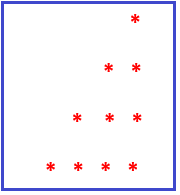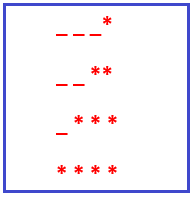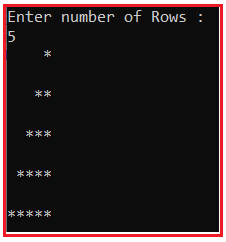# Mirrored Right Angle Triangle Star Pattern in C#

## Mirrored Right Angle Triangle Star Pattern Program in C#

In this article, I am going to discuss Mirrored Right Angle Triangle Star Pattern Program in C# with Examples. Please read our previous article where we discussed discuss Right Triangle Star Pattern Program in C#. Let us understand this. Please have a look at the below image which shows the Mirrored Right Angle Triangle Star Pattern.##### Understanding the Mirrored Right Angle Triangle Star Pattern

If we want to create Mirrored Right Angle Triangle Star Pattern with four rows, then

1. In the first row, we have 3 blank spaces and one star at the end.
2. In the second row, we have 2 blank spaces and two stars at the end.
3. In the third row, we have 1 blank space and three stars at the end.
4. In the fourth row, we have 0 blank spaces and 4 stars at the end.1. From the above pattern, it is clear that at each row number of stars is increasing +1 at each row.
2. For example, the 1st row has 1 star, the 2nd row has 2 stars and the 3rd row has 3 stars, and so on.
3. From the above pattern, it is clear that at each row number of blank spaces are decreasing –1 at each row. For example, the 1st row has 4 spaces, the 2nd row has 3 spaces, the 3rd row has 2 spaces, and so on.
##### How to Implement the Mirrored Right Angle Triangle Star Pattern in C#?

To solve the above pattern,

1. We are going to use three for loops. One outer for loop and two inner for loops.
2. The outer for loop will be used to handle the rows one by one and the two inner for loops will be used to handle the columns one by one.
3. From the above explanation, it is clear that the number of stars in each row is equal to the current row.
4. 1st row has 1 star, 2nd row has 2 stars, and so on.
5. The inner loop will be used to print the stars according to row and the outer loop job is to go on a new line after printing the current row stars.
##### C# Program to Print Mirrored Right Angle Triangle Star Pattern

The following C# Program will allow the user to input the number of rows and then print the mirrored right angle triangle star pattern on the console.

```using System;
public class MirroredRightAngleTriangleStar
{
public static void Main()
{
Console.WriteLine("Enter number of Rows :");

for (int i = 1; i <= rows; i++)
{
//rows - i  //let rows = 5
/* first row (5-1) = 4 spaces
* second row (5-2) = 3 spaces
* third row (5-3) = 2 spaces
* and so on
*/

//calculate blank space for each row one by one
for (int j = rows - i; j >= 1; j--)
{
Console.Write(" ");
}

//calculate no. of stars in each row
for (int k = 1; k <= i; k++)
{
Console.Write("*");
}
Console.WriteLine("\n");
}